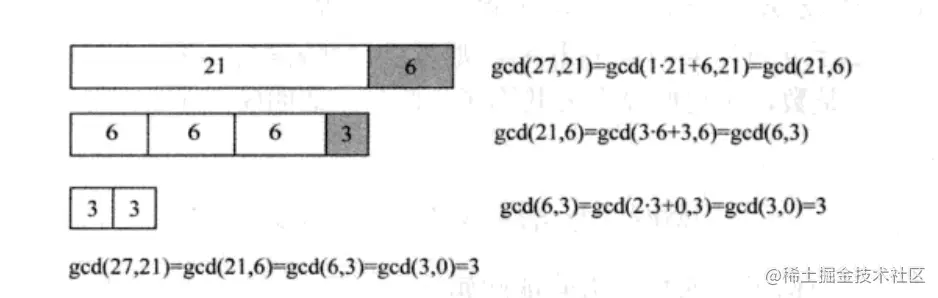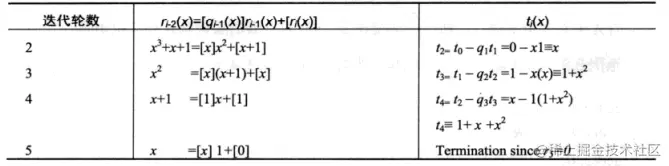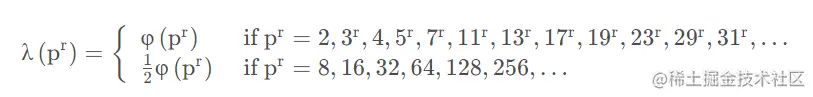# 公钥密码学中的简单数学基础

(67) 2023-10-15 09:01:01

# 公钥密码学中的简单数学基础

## 逆元

### 介绍

$a \in Z_m$
，乘法逆元
$a^{-1}$

$a·a^{-1}≡1 \pmod{m}$

$b/a≡b·a^{-1} \pmod{m}$

### 判断

$gcd(a,m)=1$
，一个元素
$a \in Z$

$a^{-1}$
，即两数互质。

### 应用

$gcd(e,\varphi(n))=1$
，是为了保证 e 的逆元存在，即保证私钥始终存在，
$d·e\equiv 1\bmod \varphi(n)$

## 欧拉定理

$a$

$m$

$gcd(a,m)=1$
，则
$a^{\varphi(m)}≡1\pmod{m}$

$\mathbb{Z}_m$

### 补充

• 对质数
$p$

$\phi(p)=p-1$
• 带有幂次素数的欧拉函数：若
$p$
为素数，且有
$n=p^r$
,则
$\varphi(n)=\varphi(p^r)=p^{r-1}\varphi(p-1)$
【易于证明将其中p倍数都去除即可】

• $m=pq$
，若
$p$

$q$
互素且大于1，则
$\varphi(m)=\varphi(p)\varphi(q)$
【易于证明因为两者互素】

### 应用

$2^{1000000 }\pmod{7}$

$2^{\varphi(7)}≡2^6≡1 \pmod{7}$

$2^{1000000 }≡(2^6)^{166666}·2^4≡2 \pmod{7}$

## 费马小定理

$a$

$p$

$a^{p}≡a\pmod{p}$

$p$

$\varphi(p)=p-1$

$a^{\varphi(p)}=a^{p-1}≡1\pmod{m}$

## 欧几里得算法

### 介绍

$r_0$

$r_1$

$gcd$

$gcd(r_0,r_1)$

$gcd$
，那就是欧几里得算法。

### 原理

$gcd(r_0,r_1)=gcd(r_0-r_1,r_1)$

$gcd(r_0,r_1)=g$
，由于g可以同时除
$r_0$

$r_1$
，则可以记为
$r_0=g·x$

$r_1=g·y$
，其中
$x>y$

$gcd(r_0-r_1,r_1)=gcd(g·(x-y),g·y)=g$

### 运算

$gcd(r_0,r_1)=gcd(r_0-r_1,r_1)=gcd(r_0-2r_1,r_1)=gcd(r_0-3r_1,r_1)=\dots=gcd(r_0-mr_1,r_1)$

$gcd(r_0\ mod\ r_1,r_1)$

$gcd(r_1,r_0\ mod\ r_1)$

$gcd(r_l,0)=r_l$
，而这实际上也就是原始问题的答案

$gcd(27,21)$image-20220925171519247

$gcd$

$gcd$

## 拓展欧几里得算法(EEA)

### 思路

$r_i$

$r_1=s_i·r_0+t_i·r_1$

$r_1=gcd(r_0,r_1)=s_i·r_0+t_i·r_1=sr_0+tr_1$

$s_l$

$s$
，同时
$t_l=t$

$r_0=973$

$r_1=301$

i
$r_{i-2}=q_{i-1}+r_i$

$r_{i}=[s_{i}]r_0+[t_i]r_1$
1
$973=3·301+70$

$70=r_0+[-3]r_1$
2
$301=4·70+21$

$21=301-4·70=r_1-4(1r_0-3r_1)=[-4]r_0+r_1$
3
$70=3·21+7$

$7=70-3·21=(1r_0-3r_1)-3(-4r_0+13r_1)=r_0+[-42]r_1$
4
$21=3·7+0$

$gcd(973,301)=7$

$s=13$

$t=-42$

### 应用

#### 计算线性组合

$gcd(r_0,r_1)=s·r_0+t·r_1$

#### 求逆元

EEA在非对称密码学中的主要应用就是计算整数的逆元

$r_1 \ mod \ r_0$

$(r_1

$gcd(r_0,r_1)=1$

$s_i·r_0+t_i·r_1=1=gcd(r_0,r_1)$

$s·r_0+t·r_1=1$

$s·0+t·r_1≡1 \ mod\ r_0$

$r_1·t≡1 \ mod\ r_0$

$t$

$r_1$

$t=r_1^{-1}\ mod \ r_0$

$a^{-1}\ mod \ m$
，直接使用输入参数为
$m$

$a$

$12^{-1}\ mod \ 67$

$i$

$q_{i-1}$

$r_i$

$s_i$

$t_i$
2 5 7 1 -5
3 1 5 -1 6
4 1 2 2 -11
5 2 1 -5 28

$12^{-1}≡28 \ mod\ 67$

$t=t+r_0$

#### 计算伽罗瓦域内的乘法逆元

$GF(2^m)$

$A(x)$

$P(x)$
，由于
$P(x)$

$gcd$

$s(x)P(x)+t(x)A(x)=gcd(P(x),A(x))=1$

$t(x)≡A^{-1}(x)\ mod \ P(x)$

$P(x)=x^3+x+1$

$GF(2^3)$

$A(x)=x^2$

$t(x)$

$t_0(x)=0$

$t_1(x)=1$image-20220925234151438

$A^{-1}(x)=t(x)=t_4(x)=x^2+x+1$

## 中国剩余定理

### 分析

$x_1$
,
$x_2$
,
$x_3$
，使得：

• $x_1$
除以3余2、除以5余0、除以7余0

• $x_2$
除以3余0、除以5余3、除以7余0

• $x3$
除以3余0、除以5余0、除以7余2

$x=x_1+x_2+x_3$
，就很容易验证这时的
$x$

$x_1$
,
$x _2$
,
$x_3$

$y_1$

$y_1$

$x_1=2\ × \ y_1$
，就很容易验证这时的
$x_1$

• 问题1-1为：寻找整数
$y_1$
满足
$y_1$
除以3余1、除以5余0、除以7余0

• 问题1-2为：寻找整数
$y_2$
满足
$y_2$
除以3余0、除以5余1、除以7余0

• 问题1-3为：寻找整数
$y_3$
满足
$y_3$
除以3余0、除以5余0、除以7余1

$y_1$
,
$y_2$
,
$y_3$
，那么就可以取
$x=2×y_1+3×y_2+2×y_3$

$z$

$z$

$35k ≡1 \pmod{3}$
，此时k就是5×7模3的逆，记作
$[35^{-1}]_3$
，那么
$z=5×7×[(5×7)^{-1}]_3$

$x=2×(5×7×[(5×7)^{-1}]_3)+3×(3×7×[(3×7)^{-1}]_5)+2×(3×5×[(3×5)^{-1}]_7)$

$X,Y$

$X−Y$

$X−Y$

$X−Y$

$3×5×7=105$

### 一般化描述

$m_1,m_2,...,m_n$

$k$

$a_1,a_2,...,a_n$
，同余式组

\left\{ \begin{aligned} x & ≡ a_1 \pmod{m_1} \\ x & ≡ a_2 \pmod{m_2} \\ \dots\\ x & ≡ a_n \pmod{m_n} \end{aligned} \right.

$N=\prod \limits_{i=1}^nm_i$

$x≡ \sum ^n_{i=1}a_i× \frac{N}{m_i}×[(\frac{N}{m_i})^{-1}]_{m_i}$

### 特殊情况

$p$

$q$

$n=p⋅q$
，则对于任意的一对
$(x_1,x_2)$

$0≤x_1

$0≤x_2
，存在唯一的数
$x$

$0≤x

$x_1=x \ mod \ p$
,且
$x_2=x \ mod\ q$
，所以任意整数
$x$

$(x_1,x_2)$

### 应用

#### 2.CRT加速模运算

$2^{1000000 }\pmod{77}$

$\varphi(77)=\varphi(7)\varphi(11)=60$

$2^{60}≡1\pmod{77}$

$2^{1000000}=(2^{60})^{16666}·2^{40}≡2^{40}\pmod{77}$

$40=2^3+2^5$

• $n_0=0$
.计算
$a_0=a≡1$

$b_1≡b^2≡4 \pmod{77}$

• $n_1=0$
.计算
$a_1=a_0≡1$

$b_2≡b_1^2≡16 \pmod{77}$

• $n_2=0$
.计算
$a_2=a_1≡1$

$b_3≡b_2^2≡25 \pmod{77}$

• $n_3=1$
.计算
$a_3=a_2b_3≡1$

$b_4≡b_3^2≡9 \pmod{77}$

• $n_4=0$
.计算
$a_4=a_3≡25$

$b_5≡b^2_4≡4 \pmod{77}$

• $n_5=1$
.计算
$a_5=a_4b_5≡23\pmod{77}$

$2^{1000000 }≡23\pmod{77}$

$b_1$

$b_2$

$x=2^{1000000 }$
.因为77=7*11，所以
$x \pmod{77}$

\left\{ \begin{aligned} x & ≡ b_1 \pmod{m_1} \\ x & ≡ b_2 \pmod{m_2} \end{aligned} \right.

$2^{\varphi(7)}≡2^6≡1 \pmod{7}$

$b_1=2^{1000000 }≡(2^6)^{166666}·2^4≡2 \pmod{7}$

$2^{\varphi(11)}≡2^{10}≡1 \pmod{11}$

$b_2=2^{1000000 }≡(2^{10})^{10000}≡1 \pmod{11}$

$x≡2×(11×[11^{-1}]_7)+1×(7×[7^{-1}]_{11})≡100≡23 \pmod{77}$

$2^{1000000 }≡23 \pmod{77}$

#### 3.RSA加密优化-CRT表示法中的运算

$(n,d)$

$c$

$m=c^d \ mod \ n$
。私钥指数
$d$

$e$

$k$

$n$
，对应的私钥指数
$d$

$k$

$m=c^d \ mod \ n$
，由于模幂的运算量随着模的比特数k的立方增加而增加。所以做两次幂运算mod p和mod q，比做一次幂运算mod n效率要高。

$p,q\ (p>q)$

• $dP=e^{−1}\ mod\ (p−1)$

• $dQ=e^{−1}\ mod\ (q−1)$

• $qInv = q^{-1} mod \ p$

$x$

$(c^d \ mod \ p, c^d \ mod \ q)$
，那么通过CRT我们知道存在唯一的值
$c^d \ mod \ n$

$[0,n-1]$

$(x_1,x_2)$

$x$
，使用Garner's方程式。

• $x=x_2+h·q$

• $h=((x_1−x_2)(q^{−1}\ mod \ p)) mod\ p$

$qInv = q^{-1} mod \ p$

$c^d\ mod\ p$

$c^d\ mod\ q$

##### 对RSA运算而言

• $dP=e^{−1}\ mod\ (p−1)= d\ mod\ (p−1)$

• $dQ=e^{−1}\ mod\ (q−1)=d\ mod\ (p−1)$

• $m_1=c^{dP} \pmod{p}$

• $m_2=c^{dQ} \pmod{q}$

• $qInv = q^{-1} mod \ p$

• $h=qInv·(m_1−m_2)\ mod\ p$

• $m=m_2+h·q$

## 裴蜀定理

### 描述

$a,b$

$gcd (a,b)=d$
，那么对于任意的整数
$x,y,ax+by$

$d$

$x,y$
，使
$ax+by=d$

### 推论及应用

• $a,b$
互质的充要条件是存在整数
$x ，y$
使
$ax+by=1$
.
• 对于裴蜀等式
$ax+by=c$
，当且仅当m是a及b的最大公约数d的倍数时有整数解。裴蜀等式有解时必然有无穷多个整数解，每组解x,y 都称为裴蜀数，可以拓展欧几里得算法获得。例如：12 和 42 的最大公因數是 6，则方程
$12x+42y=6$
有解。有
$(-3)×12+1×42=6$

$4×12+(-1)×42=6$
• 裴蜀等式也可以用来给最大公约数定义：
$d$
其实就是最小的可以写成
$ax+by$
形式的正整数。

## Carmichael定理

$a^m≡1 \pmod {n}$

$m$
，其中
$(a,n)=1$
，将
$m$

$\lambda(n)$
。在抽象代数术语中，
$\lambda(n)$

$n$

Carmichael函数也被称为规约函数(reduced totient function)以及最小泛指数函数(least universal exponent function)。

$\lambda(8)=2$
，即
$m=2$

$a^m≡1 \pmod {n}$

$a$

$(a,8)=1$
，有
$a^2=1\pmod8$

$1^2\equiv1\bmod8$

$3^2=9\equiv1\bmod8$

$5^2=25\equiv1\bmod8$

$7^{2}=49 \equiv 1\bmod 8$

#### 与欧拉函数的关系

$\varphi(8)=4$
，因为欧拉函数只需要满足对于所有与8互素的数
$a$

$a^{4} \equiv 1(\bmod 8)$
,而不需要满足
$a$

#### Carmichael定理

Carmichael定理解释了如何计算素数幂
$p^r$

$\lambda(p^r)$
。对于奇数素数的幂以及 2 和 4，
$\lambda(p^r)$

$\varphi(p^r)$
,对于
$2^3$image-20220914003446044

#### 用Carmichael定理计算 $\lambda(n)$

$n>1$

$n=p_{1}^{r_{1}} p_{2}^{r_{2}} \cdots p_{k}^{r_{k}}$
,其中，
$p_{1}

$r_{1}, r_{2}, \ldots, r_{k}$

$\lambda(n)$

$λ$

$\quad λ(n)=lcm(λ(p_1^{r1}),λ(p_2^{r2}),…,λ(p_k^{rk}))$

#### Carmichael函数的性质

$\quad a|b \Rightarrow \lambda(a)| \lambda(b)$

$\quad \lambda(\operatorname{lcm}(a, b))=\operatorname{lcm}(\lambda(a), \lambda(b))$

## 二项式定理展开

$(1+x)^n=\sum^n_{k=0} C^k_nx^k(\ mod\ n^2)=1+nx(\ mod\ n^2)$
【从
$n^2$

$n^2$

$(1+x)^n(mod\ n^2)$

$(1+x)^n=\sum^n_{k=0} C^k_nx^k(\ mod\ n^2)=1+nx(\ mod\ n^2)$

$(1+kn)^m\equiv knm+1 \pmod{n^2}$

《深入浅出密码学》

《信息安全数学基础》-上海交通大学

THE END